# Algebra chapter 11 exercise 11.2 solutions Ncert Maths

Class 6 chapter 11 Algebra exercise 11.2 solutions  Maths Ncert textbook are given.

Study the textbook lesson Algebra very well

Observe the example problems and solutions given in the solutions.

Observe the solutions and try them in your own method.

You can also see

Ncert Maths Solutions for Class 6

Algebra

Exercise 11.1

Exercise 11.2

Exercise 11.3

M

# Algebra exercise 11.2 class 6 Ncert Maths solutions

Chapter 11

Algebra

Exercise 11.2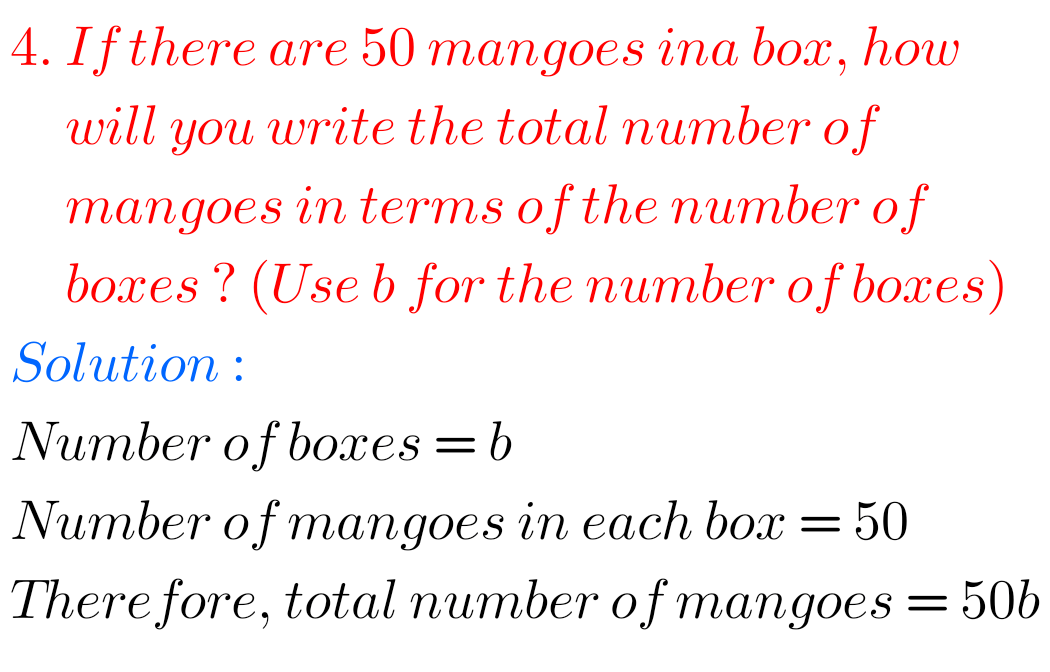F## Chapter 11 exercise 11.2 class 6 maths Ncert solutions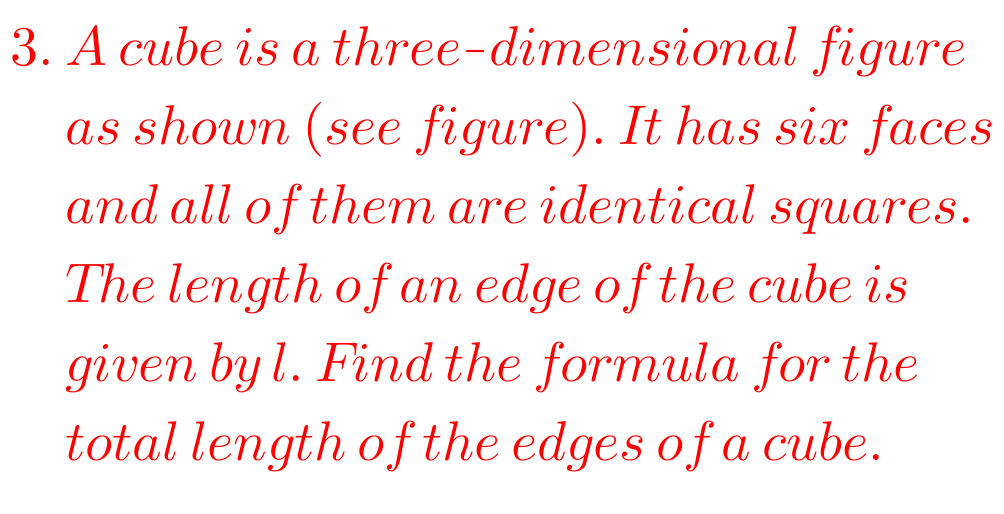F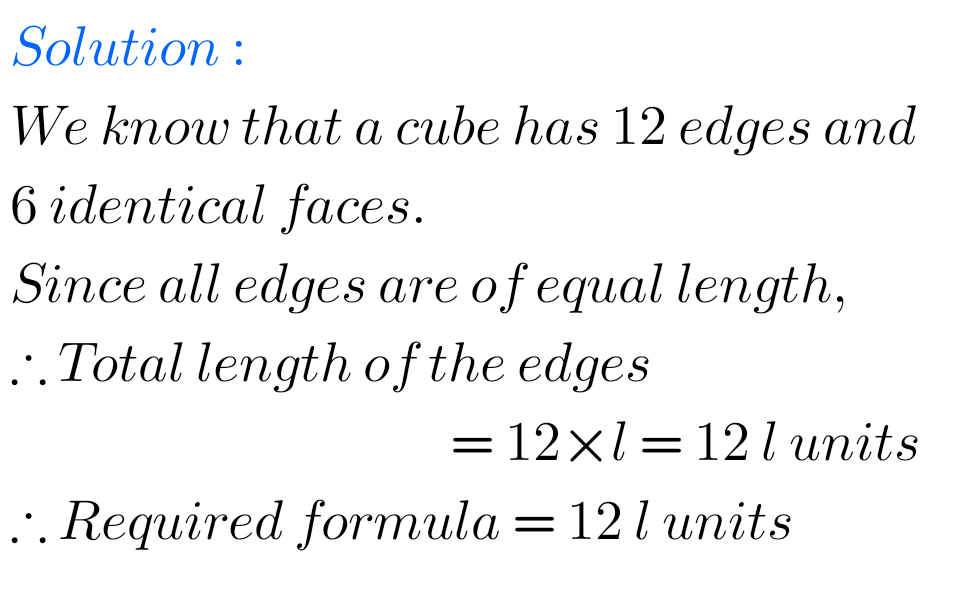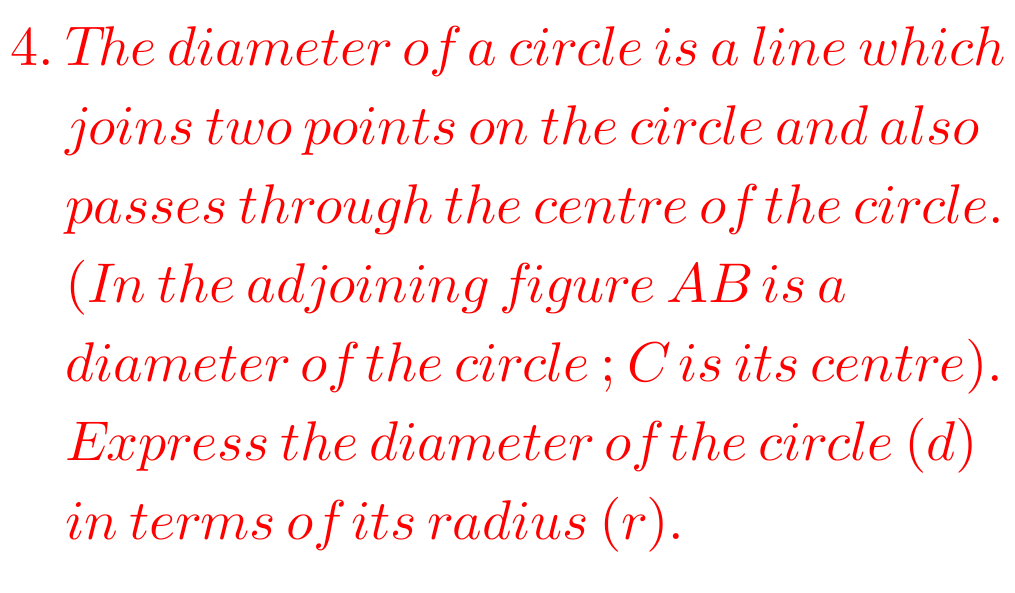F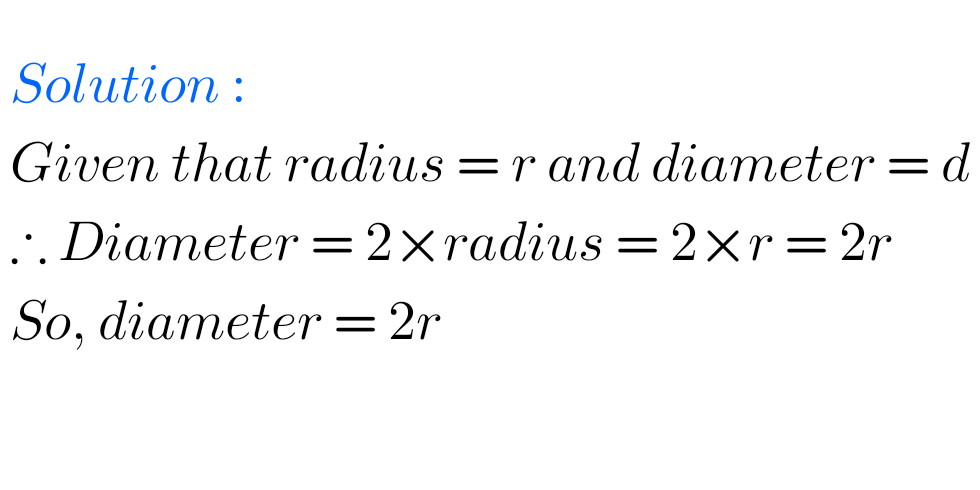## Exercise 11.2 solutions class 6 Ncert MathsM

Note : Observe the solutions and try them in your own method